Dimensions - Glossary

The Shape of Space Curriculum Materials

annulus
a tube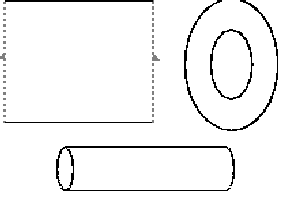Each of the above is an annulus: a gluing diagram of an annulus, a ring, and a tube.

boundary
the border of a region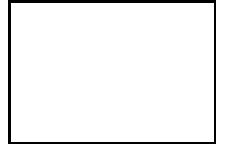The above box has one boundary that runs along all four sides.

edge
the border between two connected faces of a polyhedron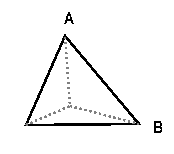In the above picture, segment AB is an edge of the tetrahedron.

finite
not infinite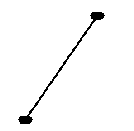The above segment is finite. It does not extend forever in any direction.

fundamental domain
the smallest part of a pattern which can be repeated to form the entire pattern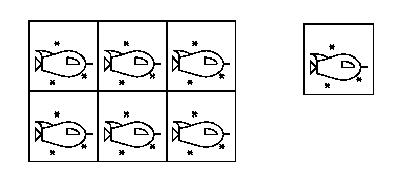In the above picture, the small square on the right with its contents is the fundamental doman for the tiling on the left.

geometry
the study of the measurements of lengths, angles, area, and volumes; the study of the relationships between points, lines, angles, surfaces, and solids. Some of these change when a surface is deformed.

glide
translation; the transformation of an image by a distance and a directionThe square on the left is the orignal square, called the preimage. The image to its right is formed by moving the preimage in the direction of the arrow. The total distance which the image is moved is equal to the length of the arrow. Note that the arrow is there for demonstrative purposes only; it is not part of the preimage.

glide-reflection
a composition of a glide and a reflection; a translation and a reflection of an object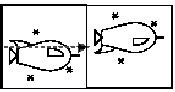Here, the preimage on the left is first reflected across the arrow and then a glide is applied to it (see glide), giving the image to the right.

gluing diagram
a diagram with connected edges marked with an arrow for gluing.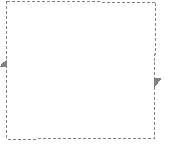This is a gluing diagram of a Mobius strip. If one were to cut it out,stretch it, and glue the sides together so that the arrows lined up, it would look like the representation of a Mobius strip in three dimensional space. This Mobius strip diagram has the same intrinsic topology of a Mobis strip placed in three dimensional space.

infinite
without endThe ray is infinite in the direction of the arrow.

reflection
mirror image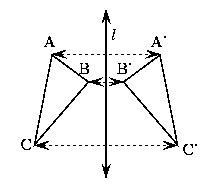Triangle ABC is reflected about line l to make triangle A'B'C'.

rotation
a spin around a certain point, called the center of rotation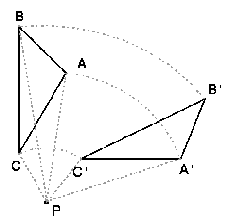Triangle ABC is rotated (45 degrees) about point P to make triangle A'B'C'

slope
the upward or downward slant of a line or the inclination of the slant of a line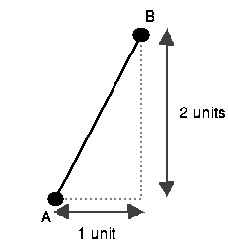Segment AB extends up two units for every unit to the right that it extends. Thus, it has slope 2/1.

symmetrical
correspondence in size, shape, and relative position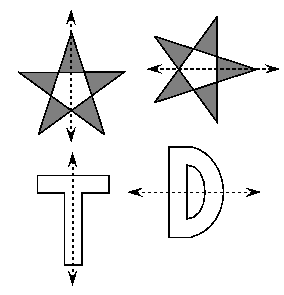Each of the above shapes is symmetrical since the part of the shape on one side of the arrow is the same as the part of the shape on the other side of the arrow except for its position.

topology
the study of surfaces; the study of properties which do not change under certain transformations

torus
the surface of a donut; can also be represented by a flat gluing diagram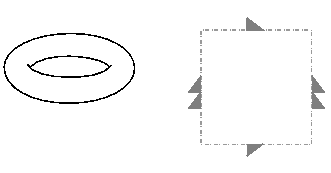Above is a doughnut-shaped torus and a torus gluing diagram. They are both intrinsically topologically a torus.

transformation
a change like a glide, rotation, reflection, or glide-reflectionThe above rotation and reflection are examples of transformations